# How to COUNTIF with non-contiguous range in Excel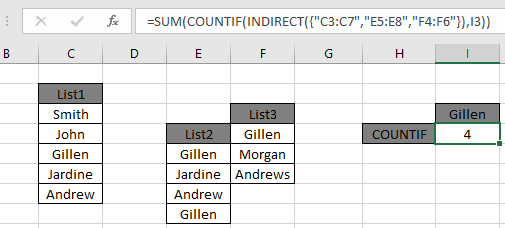In this article, we will learn How to COUNTIF with non-contiguous range in Excel.

Scenario:

COUNTIF counts cells on the basis of matched results but it doesn't take multiple ranges or arrays. Users usually combine all data values into one array or range and calculate the count of values matching using a simple COUNTIF function. As I heard a lot about facing this problem from most of the excel users. Here is the solution to it. In simple words, Consider a scenario in which we are required to count specific values in multiple arrays. For this, we can use a formula for each range and then get the sum of each result to get the count of specific values in non-contiguous ranges. But we will use a single formula to get the result.

Problem?

We have a situation where we need to use a single formula to calculate the count of matched values in multiple non-contiguous ranges or values in different arrays. We can get the same using a Countif formula for each range. That formula would be =COUNTIF(range, criteria).

How to solve the problem

There are two different approaches to get this task done. Firstly, We will use the INDIRECT function to merge all the required ranges to include. So here we will make a formula using the Three functions.

The above functions gets the task done. As the INDIRECT function feeds the COUNTIF function all the lists where values needs to be matched with criteria value. COUNTIF matches criteria and SUM function returns the total count of values.

Generic formula:

 = SUM ( COUNTIF ( INDIRECT ( { "list1" , "list2" , "list3" , ... } ) , criteria ) )

list1, list2 & list3 .. are named ranges for the multiple lists to be matched.

criteria : value or condition to match.

Example :

All of these might be confusing to understand. Let's understand how to use the function using an example. Here we have 3 lists having some names and we need the count or times the name "Gillen" is repeated.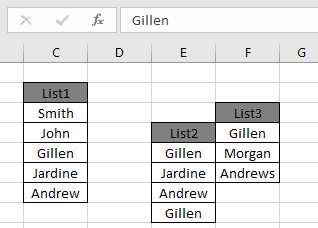The different list values are given named ranges as list1, list2 & list3. Utilize the below given formula to get the count.

Use the formula:

 = SUM ( COUNTIF ( INDIRECT ( { "C3:C7" , "E5:E8" , "F4:F6" } ) , I3 ) )

Explanation:

• INDIRECT({"C3:C7", "E5:E8", "F4:F6"}) evaluates text values and feeds the ranges to the COUNTIF function.
• COUNTIF function returns each count for different ranges matching all the lists with value in I3 cell.
• SUM function returns the sum after adding all the counts.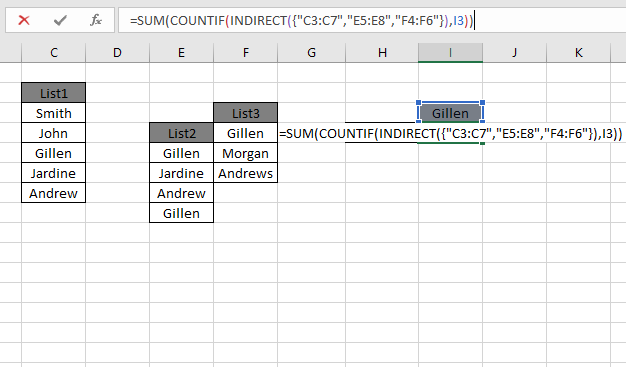This is how a formula looks like in the cell. Press Enter to get the count. Here the values satisfying the condition in I3. There matched values must return count for each individual as 1 from list1, 2 from list2 & 1 again from list3 and result be 4.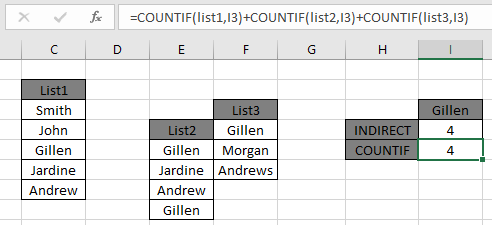As you can see in the above snapshot the above explained COUNTIF formula returns the count of cells with non-contiguous range in Excel.

You could perform the individual count of each range as shown in below formula and snapshot.

Use the formula:

 = COUNTIF ( list1, I3 ) + COUNTIF ( list2, I3 ) + COUNTIF ( list3 , I3 )

Explanation:

• Multiple COUNTIF function returns each count for different ranges matching all the lists with value in I3 cell.
• + operator returns the sum after adding all the counts.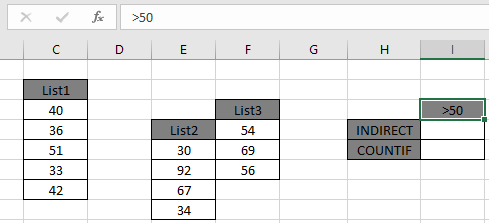As you can see in the above snapshot the above explained COUNTIF formula returns the count of cells with non-contiguous range in Excel.

The above examples were performed over Text values. So to get the same operation performed over numbers

Here we have 3 lists having numbers and we need the count the numbers having condition. Condition numbers greater than 50.The different list values are given named ranges as list1, list2 & list3. Utilize the below given formula to get the count.

Use the formula:

 = SUM ( COUNTIF ( INDIRECT ( { "C3:C7" , "E5:E8" , "F4:F6" } ) , I3 ) )

Explanation:

• INDIRECT({"C3:C7", "E5:E8", "F4:F6"}) evaluates values and feeds the ranges to the COUNTIF function.
• COUNTIF function returns each count for different ranges matching all the lists with condition in I3 cell.
• SUM function returns the sum after adding all the counts.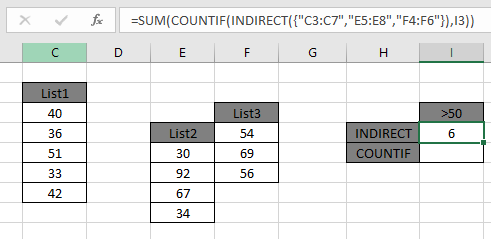This is how a formula looks like in the cell. Press Enter to get the count. Here the numbers satisfying the condition greater than 50. There are { 51, 92, 67, 54, 69, 56 } values which needed to matched and result be 6.As you can see in the above snapshot the above explained COUNTIF formula returns the count of cells with non-contiguous range and specified condition in Excel.

You could perform the individual count of each range as shown in below formula and snapshot.

Use the formula:

 = COUNTIF ( list1, I3 ) + COUNTIF ( list2, I3 ) + COUNTIF ( list3 , I3 )

Explanation:

• Multiple COUNTIF function returns each count for different ranges matching all the lists with condition in I3 cell.
• + operator returns the sum after adding all the counts.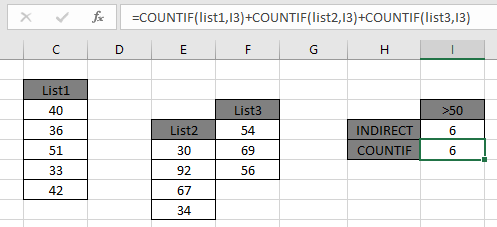As you can see in the above snapshot the above explained COUNTIF formula returns the count of cells with non-contiguous range in Excel.

NOTE:

You must be wondering why this task can't be done using COUNTIFS function or SUMPRODUCT function. COUNTIFS function uses AND operator with returned values but we needed OR operator permoing over values. SUMPRODUCT function has a specific condition before using that different lists must have the same number of elements or values.

Here are all the observational notes using the formula in Excel
Notes :

1. wildcards help with extracting value from the substrings.
2. The COUNTIF function supports logical operators like <, >, <>, = but these are used using double quote sign ( " ) with arguments. But if you are using cell reference for the criteria quotes can be ignored.
3. Criteria argument should use quote sign (") for texts ( like "Gillen" ) or numbers ( like ">50" ).
4. The formula can be used for both texts and numbers.

Hope this article about How to COUNTIF with non-contiguous range in Excel is explanatory. Find more articles on calculating values and related Excel formulas here. If you liked our blogs, share it with your friends on Facebook. And also you can follow us on Twitter and Facebook. We would love to hear from you, do let us know how we can improve, complement or innovate our work and make it better for you. Write to us at info@exceltip.com.

Related Articles :

How to use the SUMPRODUCT function in Excel: Returns the SUM after multiplication of values in multiple arrays in excel. This function can be used to do multiple tasks. This is one of the most versatile functions.

COUNTIFS with Dynamic Criteria Range : To count with dynamic criteria range we simply use the INDIRECT function. This function can

COUNTIFS With OR For Multiple Criteria : Count cells having multiple criteria match using the OR function. To put an OR logic in COUNTIFS function you will not need to use the OR function.

Using the IF with AND / OR Functions in Microsoft Excel : These logical functions are used to carry out multiple criteria calculations. With IF the OR and AND functions are used to include or exclude matches.

How to use OR function in Microsoft Excel : The function is used to include all the TRUE values in multiple criterias.

How to Count Cells That Contain This Or That in Excel in Excel :To cells that contain this or that, we can use the SUMPRODUCT function. Here's how you do those calculations.

Popular Articles :